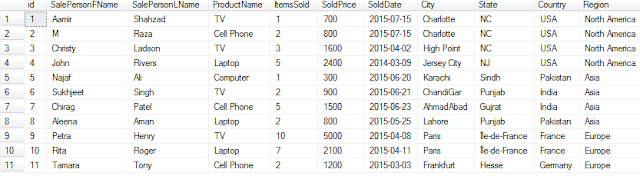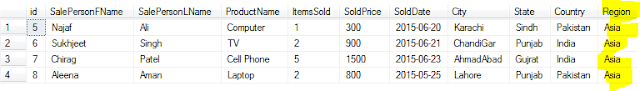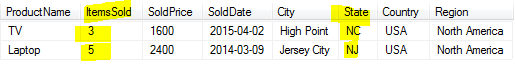### How to use Where clause in Select Statement in SQL Server - SQL Server / TSQL Tutorial Part 109

In this post we will get familiar with Where Clause in TSQL.
Where clause is used in DML statements such as select, update, delete to filter the records according to criteria we used in where clause.

Let's create the table by using this script to create TotalSale Table so we can use for different queries.

We know that to see all the data from a table we can use

`Select * from dbo.TotalSale`Select all records from a table by using Select * in SQL Server - SQL Server / TSQL Tutorial

With Where clause we can use different types of operations to filter the records such =, < >, >, >=, <, <=, IN, BETWEEN, LIKE, IS NULL or IS NOT NULL.

Let's try few of above operators with Select query for dbo.TotalSale table.

1) Get all the records from dbo.TotalSale table for Region equal to Asia.
```
```
```Select * From dbo.TotalSale
where Region='Asia'```
```
```
```
```How to filter records in SQL Server Select query by using Where clause
```
```
2) Get all the records from dbo.TotalSale where ItemsSold is greater than 3.
```Select * From dbo.TotalSale
where ItemsSold >3```How to get only records where value is greater than some value in SQL Server Select Query

3) Get all the records where State Name starts with N letter

```Select * From dbo.TotalSale
where State like 'N%'```
```
```How to use like operator with Where clause to filter the records in SQL Server - SQL Server Tutorial
```
```
AND and OR keywords can be used to check multiple criteria.

4) If we want to get only the records where ItemsSold is greater than 2 and State name starts with N letter.

```Select * From dbo.TotalSale
where itemssold>2
And state like 'N%'```
```
```How to use AND keyword in Where clause in Select Query in SQL Server - SQL Server / TSQL Tutorial
```
```
When you use AND, all the conditions should be true to return record.

4) Get all the records where ItemsSold is greater than 2 or state name start with N.

```Select * From dbo.TotalSale
where itemssold>2
OR state like 'N%'```
```
```How to use OR keyword in Select query - SQL Server / TSQL Tutorial
```
```
As you can see that when we used OR keyword, either of the condition has to be true to return the results.